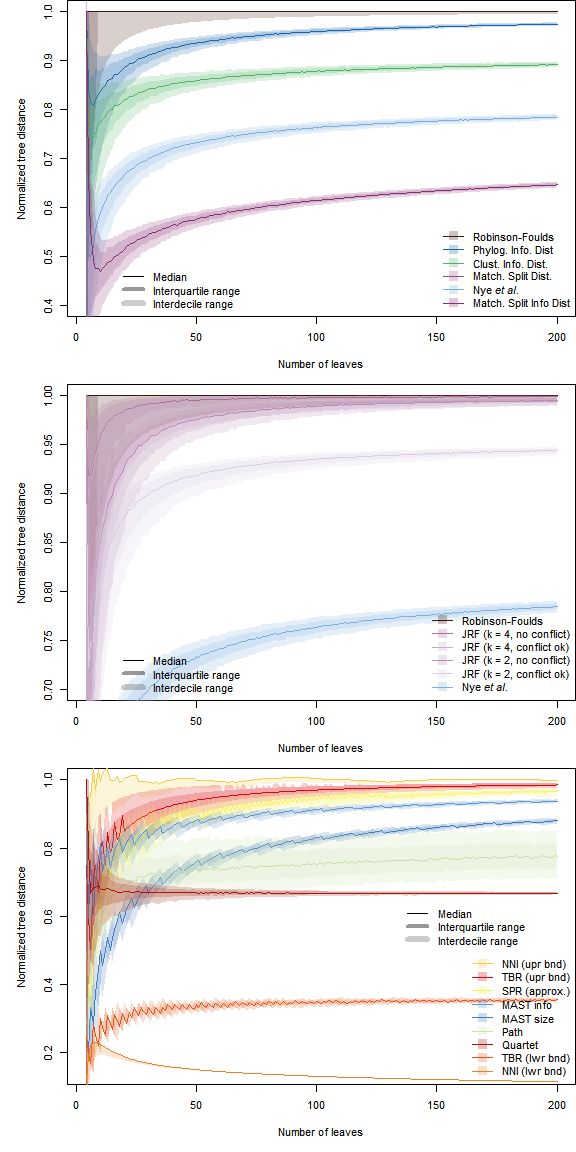# Interpreting tree distance metrics

## Expected values for random trees

Context for tree distances can be provided by comparison with the distance expected for a pair of random trees.

The figure below shows how the median normalized tree distance varies with the number of leaves in the trees being compared. 1 000 random pairs of $$n$$-leaf trees were generated for $$4 \leq n \leq 200$$, and the distances between them calculated. Shaded envelopes denote the interdecile and interquartile ranges. The raw data are available in the data object randomTreeDistances in the R package ‘TreeDistData’.Information-based metrics are normalized against the total information content of each pair of trees. The (Jaccard-)Robinson-Foulds, Nye et al., maximum agreement subtree and Quartet metrics are normalized based on the maximum possible value. The SPR distance is normalized against $$\frac{n}{2}$$; this value lies between the definitive bounds of the maximum diameter of the SPR distance (Allen & Steel, 2001), and was not exceeded by any of the random tree pairs. The TBR distance is normalized against $$n$$. The path, Matching Split Distance and NNI metrics do not have a readily calculated maximum value and are thus crudely ‘normalized’ against an approximate maximum, estimated by fitting a polynomial to the the maximum observed distance for each number of leaves. Candidate polynomial functions were evaluated based on the Akaike and Bayesian information criteria.

Note that the path and Matching Splits distances exhibit a prominently larger variability than other metrics, suggesting that they are less consistent in the score that they assign to a random pair of trees. In combination with the difficulty in calculating the range of these metrics, this makes their absolute value difficult to interpret.